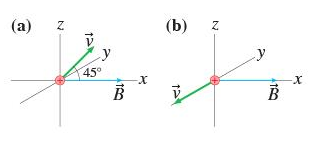# In figure (a), what is the magnetic force f⃗ on the proton? give your answers in component form

A proton moves in the magnetic field B? =0.56i^T with a speed of 1.0x107m/s in the directions shown in the figure. (Figure 1)

Part A
In Figure (a), what is the magnetic force F? on the proton?
Give your answers in component form. Express vector F? in the form of Fx, Fy, Fz, where the x, y, and z components are separated by commas.

Part B
In Figure (b), what is the magnetic force F? on the proton?
Give your answers in component form. Express vector F? in the form of Fx, Fy, Fz, where the x, y, and z components are separated by commas.